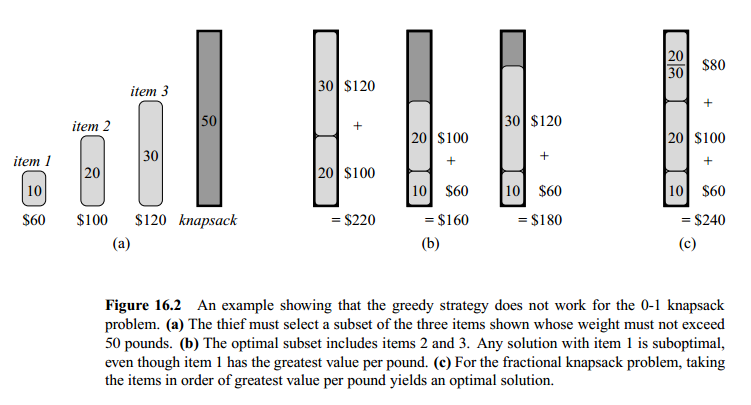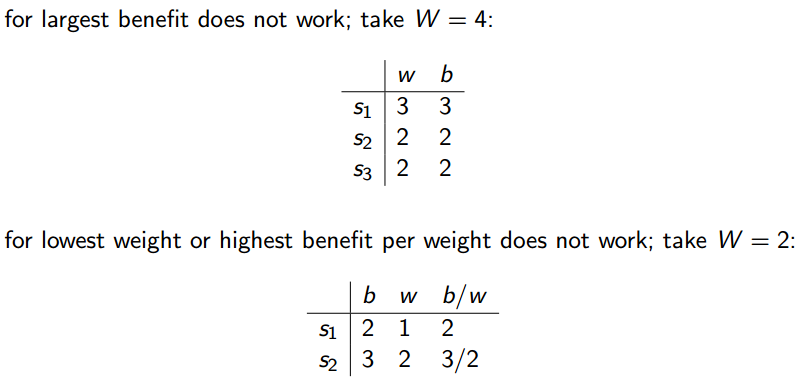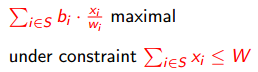# Fractional knapsack

Updated:

⚠️ This post was created when I was in high school and is no longer maintained.

In this setting, the thief can take fractions of items, rather than having to make a binary (0-1) choice for each item. You can think of an item in the 0-1 knapsack problem as being like a gold ingot and an item in the fractional knapsack problem as more like gold dust. —— CRLS

## 1. Fractional Knapsack VS 0-1 Knapsack

• Both knapsack problems exhibit the optimal-substructure property.

• For the 0-1 problem, consider the most valuable load that weighs at most W pounds.
• If we remove item j from this load, the remaining load must be the most valuable load weighing at most W - w_j that the thief can take from the n-1 original items excluding item j .
• For the comparable fractional problem,
• consider that if we remove a weight w of one item j from the optimal load, the remaining load must be the most valuable load weighing at most W - w that the thief can take from the n-1 original items plus w_j - w pounds of item j (the remains of item j).• As the Fig 16.2 in CRLS demonstrates, although the problems are similar, we can solve the fractional knapsack problem by a greedy strategy, but we cannot solve the 0-1 problem by such a strategy.

An example,## 2 Intuition of the greedy choice

• To solve the fractional problem, we first compute the value per pound v_i = b_i / w_i for each item i.

• Obeying a greedy strategy, the thief begins by taking as much as possible of the item with the greatest value per pound.

• If the supply (w_i - q_i where q_i is the quantity that we took from the total amount of item i) of that item is exhausted and we can still carry more (weightLimit > currWeight), we take as much as possible of the item with the next greatest value per pound, and so forth, until we reach our weight limit.

• Therefore, our goal lies in:(x_i here is the same concept as the q_i above)

## 3. Pseudocode

struct Item {
double benifit;
double weight;
} item;

items := Array[item]

Algorithm FractionalKnapsack(items, weightLimit):
new values[1 ~ n]
new quantities[1 ~ n]

For i := 1 to n do
quantities[i] := 0
values[i] := items[i].benifits / items[i].weight

Sort(items, key=values, desc) // or using a max-heap

currWeight := 0
For j := 0 to n do
if currWeight < weightLimit then
quantities[j] := Min(items[j].weight, availableLoad) --> make greed choice
currWeight += quantities[j]
else
break

total := Reduce(quantities)

• We could either represent the items set as a priority queue (with the highest priority for the highest benefit per weight value) using a max-heap, or
• we can sort the items in O(nlogn) time anyway;
• T(n) = O(n) + O(nlgn) + O(n) ∈ O(nlgn)